Name _____________________________Date ___________________
Isosceles Triangles
Find the value of x.

 1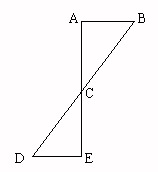Line ACBCm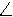DCE = 72mCBA = x
 2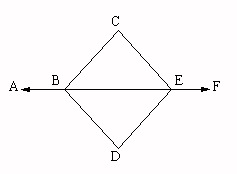mDBEmDEBmABD = 123mDBE = x
 3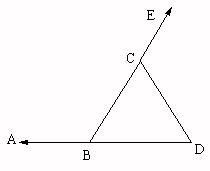Line BCCDmDCE = 3x + 16mCBD = x + 17
 4mCBEmCEBmCEB = 39mABC = x
 5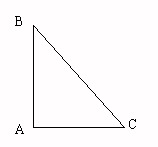Line ABACmBCA = 5x - 46mBAC = 2x + 8
 6Line BDDCmDBE = 58mABD = x
 7mABCmBCAmBAC = 4x - 24mBCA = 5x - 38
 8mCBDmCDBmCBD = 40mDCE = x
 9Line BCCDmCBE = 41mABC = x
 10mCABmCBAmDCE = 4x + 38mCBA = 3x + 31